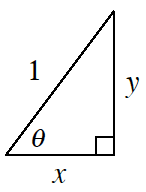### Home > GC > Chapter 11 > Lesson 11.1.4 > Problem11-57

11-57.

Multiple Choice: In the diagram at right, the value of $y$ is:

1. $\sin\theta$

1. $\cos\theta$

1. $\tan\theta$

1. $x$

1. None of theseThink about each of the trigonometric relationships.
Which one applies to this triangle?